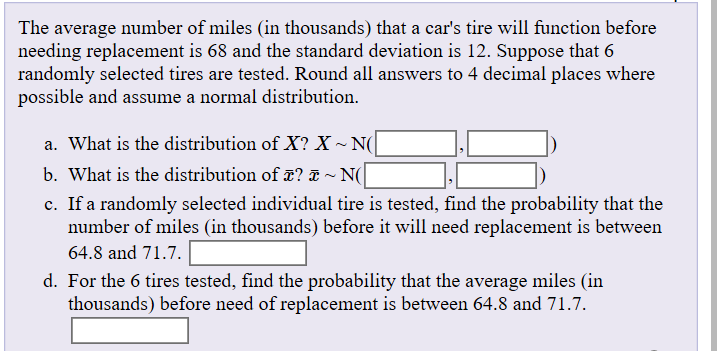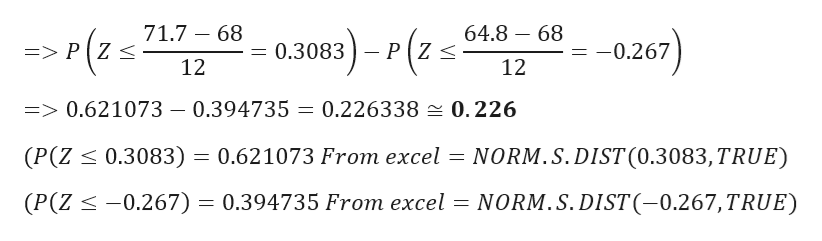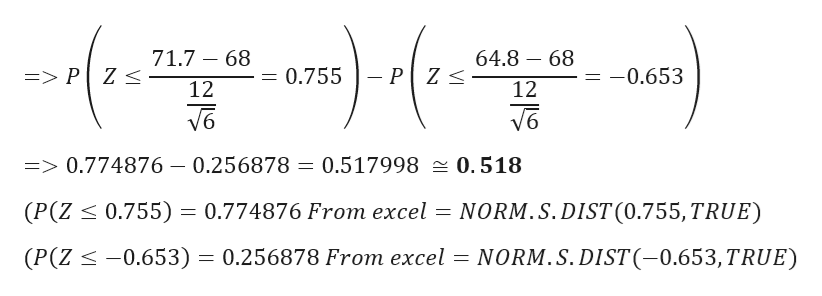# The average number of miles (in thousands) that a car's tire will function beforeneeding replacement is 68 and the standard deviation is 12. Suppose that 6randomly selected tires are tested. Round all answers to 4 decimal places wherepossible and assume a normal distribution.a. What is the distribution of X? X~ N(b. What is the distribution of T?~ N(c. If a randomly selected individual tire is tested, find the probability that thenumber of miles (in thousands) before it will need replacement is between64.8 and 71.7.d. For the 6 tires tested, find the probability that the average miles (inthousands) before need of replacement is between 64.8 and 71.7.

Question
163 viewshelp_outlineImage TranscriptioncloseThe average number of miles (in thousands) that a car's tire will function before needing replacement is 68 and the standard deviation is 12. Suppose that 6 randomly selected tires are tested. Round all answers to 4 decimal places where possible and assume a normal distribution. a. What is the distribution of X? X~ N( b. What is the distribution of T? ~ N( c. If a randomly selected individual tire is tested, find the probability that the number of miles (in thousands) before it will need replacement is between 64.8 and 71.7. d. For the 6 tires tested, find the probability that the average miles (in thousands) before need of replacement is between 64.8 and 71.7. fullscreen
check_circle

Step 1

Given data

Mean = 68

Standard deviation = 12

As per binomial approximation

Mean = np > 5 and standard deviation = npq > 5

So it will follow normal distribution

a) The distribution of x is Normal i.e. X ~ N(68,122)

b) The distribution of x-bar is

Step 2

c)

If the randomly selected individual tire is tested, probability that the number of miles before it will need replacement is between 64.8 and 71.7 is given byhelp_outlineImage Transcriptionclose71.7 68 64.8 68 s)P2 - PZ < => P Z < 0.3083 -0.267 12 12 => 0.621073 - 0.394735 = 0.226338 0.226 (P(Z 0.3083) 0.621073 From excel NORM.S.DIST(0.3083, TRUE) (P(Z -0.267) = 0.394735 From excel = NORM.S. DIST(-0.267,TRUE) fullscreen
Step 3

d)

For the 6 tires tested, probability that the average miles before...help_outlineImage Transcriptionclose71.7 - 68 64.8 68 0.755 P Z => P Z < -0.653 12 12 => 0.774876 - 0.256878 0.517998 0.518 0.755) = 0.774876 From excel = NORM. S. DIST (0.755,TRUE) (P(Z (P(Z -0.653) = 0.256878 From excel = NORM.S. DIST(-0.653,TRUE) fullscreen

### Want to see the full answer?

See Solution

#### Want to see this answer and more?

Solutions are written by subject experts who are available 24/7. Questions are typically answered within 1 hour.*

See Solution
*Response times may vary by subject and question.
Tagged in

### Statistics